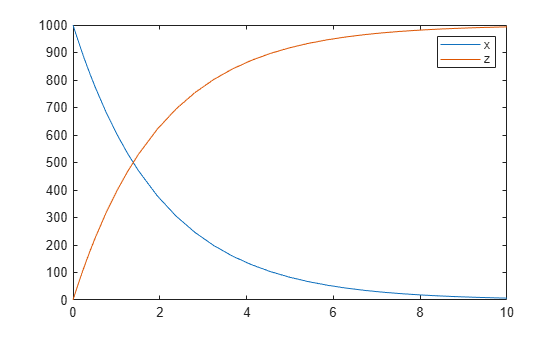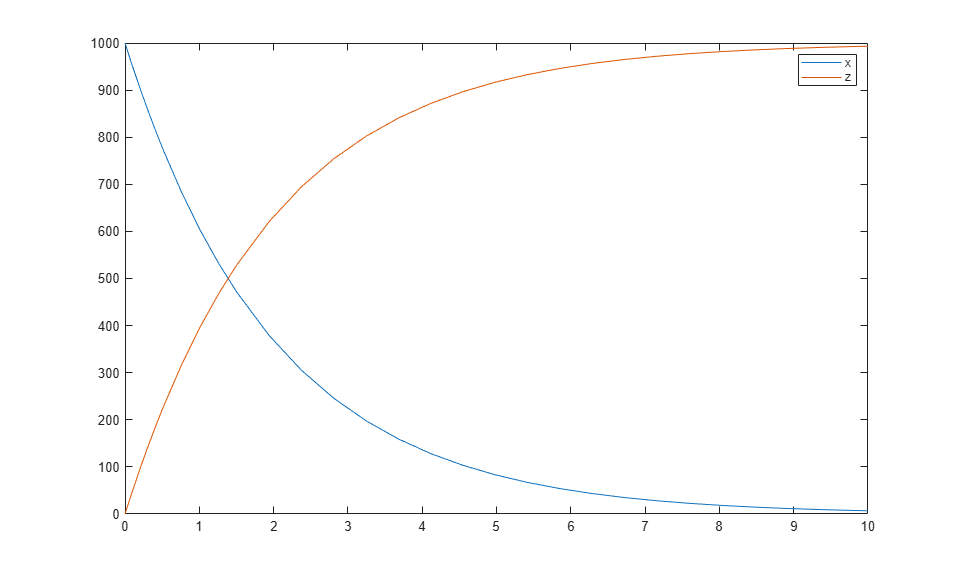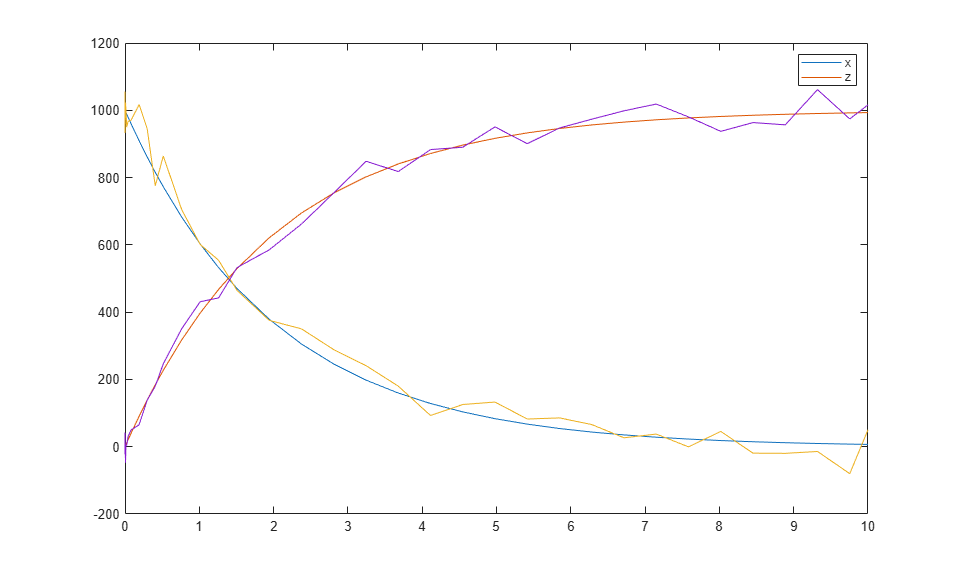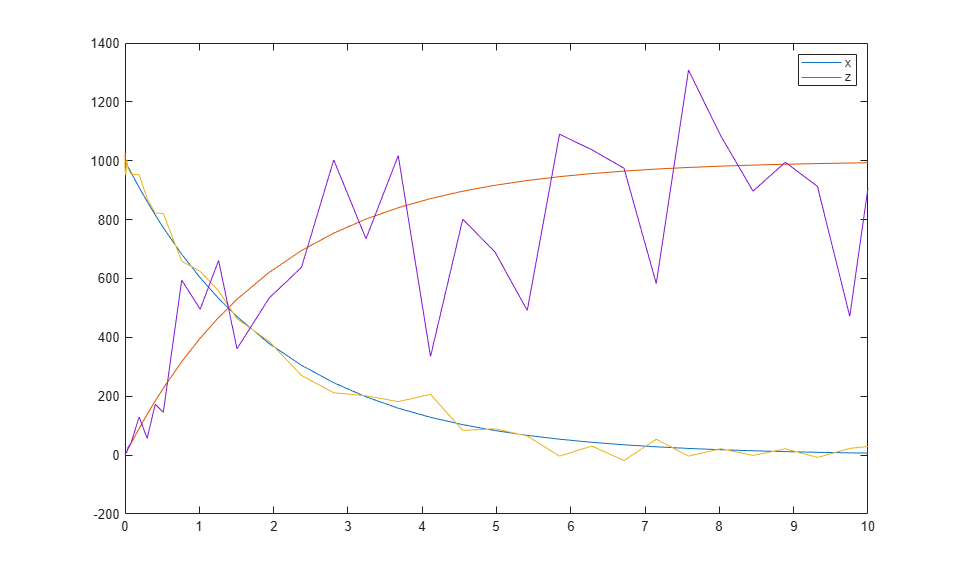Documentation

sbiosampleerror

Sample error based on error model and add noise to simulation data

Description

example

sdN = sbiosampleerror(sd,errormodel,errorparam) adds noise to the simulation data sd using one or more error models errormodel and error parameters errorparam.

Examples

collapse all

This example adds noise (or error) to the simulation data from a radioactive decay model with the first-order reaction: $\frac{dz}{dt}=c\cdot x$, where x and z are species and c is the forward rate constant.

Load the sample project containing the radiodecay model m1.

Simulate the model.

[t,sd,names] = sbiosimulate(m1);

Plot the simulation results.

plot(t,sd);
legend(names,'AutoUpdate','off');
hold onAdd noise to the simulation results using the constant error model with the error parameter set to 20.

sdNoisy = sbiosampleerror(sd,'constant',20);

Plot the noisy simulation data.

plot(t,sdNoisy);This example defines a custom error model using a function handle and adds noise to simulation data of a radioactive decay model with the first-order reaction, where x and z are species, and c is the forward rate constant.

Load the sample project containing the radiodecay model m1.

Suppose you have a simple custom error model with a standard mean-zero and unit-variance (Gaussian) normal variable e, simulation results f, and two parameters p1 and p2:Define a function handle that represents the error model.

em = @(y,p1,p2) y+p1+p2*randn(size(y));

Simulate the model.

[t,sd,names] = sbiosimulate(m1);

Plot the simulation results and hold the plot.

plot(t,sd);
legend(names,'AutoUpdate','off');
hold onSample the error using the previously defined custom function with two parameters set to 0.5 and 30, respectively.

sdNoisy = sbiosampleerror(sd,em,{0.5,30});

Plot the noisy simulation data.

plot(t,sdNoisy);You can also apply a different error model to each state, which is a column in sd. Suppose you want to apply the custom error model (em) to the first column (species x data) and the proportional error model to the second column (species z data).

hold off
sdNoisy = sbiosampleerror(sd,{em,'proportional'},{{0.5,30},0.3});
plot(t,sd);
legend(names,'AutoUpdate','off');
hold on
plot(t,sdNoisy);Input Arguments

collapse all

Simulation results, specified as a SimData object or matrix.

Error model(s), specified as a character vector, string, function handle, string vector, cell array of character vectors, or cell array containing a mixture of character vectors and function handles.

If it is a string vector or cell array, its length must match the number of columns (responses) in sd, and each error model is applied to the corresponding column in sd. If it is a single character vector, string, or function handle, the same error model is applied to all columns in sd.

The first argument of a function handle must be a matrix of simulation results. The subsequent arguments are the parameters of the error model supplied in the errorparam input argument. The output of the function handle must be a matrix of the same size as the first input argument (simulation results).

For example, suppose you have a custom error model with a standard mean-zero and unit-variance (Gaussian) normal variable e, simulation results f, and two parameters p1 and p2: $y=f+p1+p2\ast e$. You can define the corresponding function handle as follows.

em = @(y,p1,p2) y+p1+p2*randn(size(y));
where y is the matrix of simulation results and p1 and p2 are the error parameters. The output of the function handle must be the same size as y, which is the same as the simulation results specified in the sd input argument. The parameters p1 and p2 are specified in the errorparam argument.

There are four built-in error models. Each model defines the error using a standard mean-zero and unit-variance (Gaussian) variable e, simulation results f, and one or two parameters a and b. The models are:

• 'constant': $y=f+ae$

• 'proportional': $y=f+b|f|e$

• 'combined': $y=f+\left(a+b|f|\right)e$

• 'exponential': $y=f\ast \mathrm{exp}\left(ae\right)$

Error model parameter(s), specified as a scalar, vector, or cell array. If errormodel is 'constant', 'proportional', or 'exponential', then errorparam is specified as a numeric scalar. If it is 'combined', then errorparam is specified as a row vector with two elements [a b].

If errormodel is a cell array, then errorparam must be a cell array of the same length. In other words, errorparam must contain N elements, where N is the number of error models in errormodel. Each element must have the correct number of parameters for the corresponding error model.

For example, suppose you have three columns in sd, and you are applying a different error model (constant, proportional, and exponential error models with error parameters 0.1, 2, and 0.5, respectively) to each column, then errormodel and errorparam must be cell arrays with three elements as follows.

errormodel = {'constant','proportional','exponential'};
errorparam = {0.1,2,0.5};

Output Arguments

collapse all

Simulation results with added noise, returned as a vector of SimData objects or numeric matrix. If sd is a vector of SimData objects, sdN is also a vector of SimData objects, and the error is added to each column in the sd.Data property. If sd is specified as a matrix, sdN is a matrix, and the error is added to each column in the matrix.